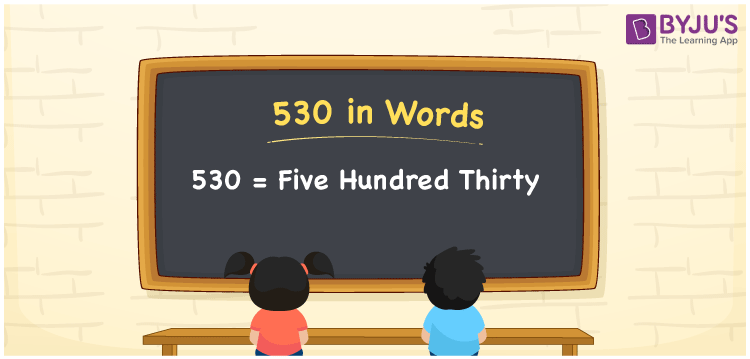# 530 in words

530 in words is written as Five Hundred and Thirty. 530 represents the count or value. The article on Counting Numbers can give you an idea about count or counting. The number 530 is a 3 digit number that is used in expressions related to money, days, distance, length, weight and so on. Let us consider an example for 530. “This player has played more than Five Hundred and Thirty cricket matches.”

 530 in words Five Hundred and Thirty Five Hundred and Thirty in Numbers 530

## 530 in English Words## How to Write 530 in Words?

We can convert 530 to words using a place value chart. The number 530 has 3 digits, so let’s make a chart that shows the place value up to 3 digits.

 Hundreds Tens Ones 5 3 0

Thus, we can write the expanded form as:

5 × Hundred + 3 × Ten + 0 × One

= 5 × 100 + 3 × 10 + 0 × 1

= 530

= Five Hundred and Thirty.

530 is the natural number that is succeeded by 529 and preceded by 531.

530 in words – Five Hundred and Thirty.

Is 530 an odd number? – No.

Is 530 an even number? – Yes.

Is 530 a perfect square number? – No.

Is 530 a perfect cube number? – No.

Is 530 a prime number? – No.

Is 530 a composite number? – Yes.

## Solved Example

1. Write the number 530 in expanded form

Solution: 5 × 100 + 3 × 10 + 0 × 1

We can write 530 = 500 + 30 + 0

= 5 × 100 + 3 × 10 + 0 × 1.

## Frequently Asked Questions on 530 in words

Q1

### How to write the number 530 in words?

530 in words is written as Five Hundred and Thirty.
Q2

### Is 530 divisible by 3?

No. 530 is not divisible by 3.
Q3

### Is 530 a prime number?

No. 530 is not a prime number.